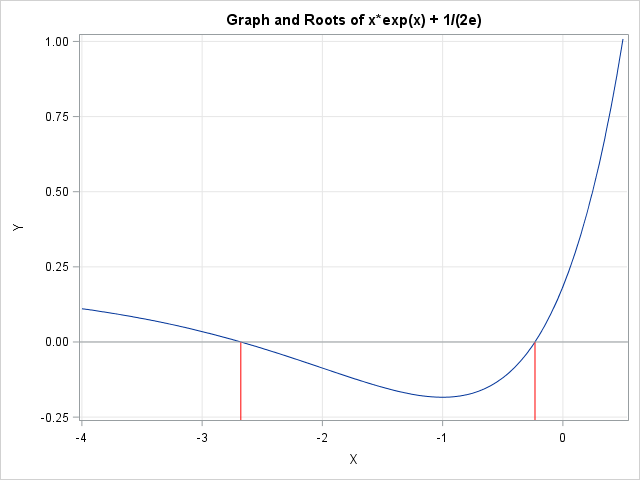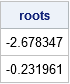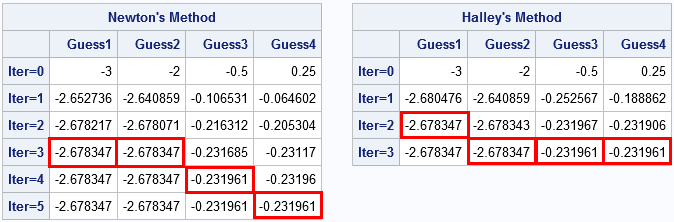Edmond Halley (1656-1742) is best known for computing the orbit and predicting the return of the short-period comet that bears his name. However, like many scientists of his era, he was involved in a variety of mathematical and scientific activities. One of his mathematical contributions is a numerical method for finding the roots of a real-valued function. The technique, which is called Halley's method, is similar to Newton's method, but converges more rapidly in the neighborhood of a root.

There are dozens of root-finding methods, but Newton's method is the gold standard for finding roots of general nonlinear functions. It converges quadratically and is easy to implement because the formula requires only the evaluation of a function and its first derivative. Other competing methods do not use derivatives at all, or they use higher-order derivatives.

Halley's method falls into the latter category. Like Newton's method, it requires an initial guess for the root. It evaluates the function and its first two derivatives at an approximate location of the root and uses that information to iteratively improve the approximation. This article compares Halley's method with Newton's method and suggests a class of functions for which Halley's method is preferable.

Halley's root-finding method: An alternative to Newton's method #SASTip
Click To Tweet

### An example of finding a root in SAS/IML

Consider the function f(x) = x exp(x). If you want to find the values of x for which f(x)=c, you can recast the problem as a root-finding problem and find the roots of the function f(x)-c. For this particular function, the equation f(x)-c has one root if c ≥ 0 and two roots if -1/e < c < 0.To be concrete, let c = -1/(2e) so that the equation has two roots. The function f(x)-c is shown to the right and the two roots are marked by red line segments. You can use the built-in FROOT function in SAS/IML to locate the roots. The FROOT function does not use Newton's method. Instead, it requires that you specify an interval on which to search for a root. From the graph, you can specify two intervals that bound roots, as follows:

```proc iml; /* The equation f(x)-c for f(x) = x exp(x) */ start func(x) global(g_target); return x # exp(x) - g_target; finish;   g_target = -1 / (2*constant('e')); /* parameter; function has two roots */ intervals = {-3 -2, /* one root in [-3,-2] */ -1 0}; /* another root in [-1,0] */ roots = froot("func", intervals); /* find roots in each interval */ print roots;```The FROOT function is effective and efficient. It always finds an approximate root provided that you can supply a bounding interval on which the function changes signs. However, sometimes you don't know a bounding interval, you only know an approximate value for the root. In that case, Newton-type methods are preferable.

### Newton's method versus Halley's method

Halley's method is an extension of Newton's method that incorporates the second derivative of the target function. Whereas Newton's method iterates the formula xn+1 = xn - f(xn) / f′(xn), Halley's method contains an extra term in the denominator:
xn+1 = xn - f(xn) / [f′(xn+1) - 0.5 f(xn) f″(xn) / f′(xn)].
Notice that if f″(xn)=0, then Halley's method reduced to Newton's method.

For many functions, the computational cost of evaluating the extra term in Halley's formula does not offset the gain in convergence speed. In other words, it is often quicker and easier to use Newton's simpler formula than Halley's more complicated formula. However, Halley's method is worth implementing when the ratio f″(x) / f′(x) can be evaluated cheaply. The current function provides an example: f′(x) = (x+1) exp(x) and f″(x) = (x+2) exp(x), so the ratio is simply (x+2) / (x+1). This leads to the following SAS/IML functions. One function implements Newton's iteration and the other implements Halley's iteration:

```/* Compute iterations of Newton's method for several initial guesses: f(x) = x#exp(x) - c */ start NewtonIters(x0, numIters=1) global(g_target); z = j(numIters+1, ncol(x0)); z[1,] = x0; do i = 2 to numIters+1; x = z[i-1,]; fx = x # exp(x) - g_target; /* f(x) */ dfdx = (1+x) # exp(x); /* f'(x) */ z[i,] = x - fx / dfdx; /* new approximate root */ end; return z; finish;   /* Compute iterations of Halley's method for several initial guesses: f(x) = x#exp(x) - c */ start HalleyIters(x0, numIters=1) global(g_target); z = j(numIters+1, ncol(x0)); z[1,] = x0; do i = 2 to numIters+1; x = z[i-1,]; fx = x # exp(x) - g_target; /* f(x) */ dfdx = (1+x) # exp(x); /* f'(x) */ R = (2+x) / (1+x); /* ratio f''(x) / f'(x) */ z[i,] = x - fx / (dfdx - 0.5*fx#R); /* new approximate root */ end; return z; finish;```

Notice that the functions are practically identical. Halley's method computes an extra term (R) and includes that term in the iterative formula that converges to the root. I wrote the function in vectorized form so that you can pass in multiple initial guesses and the functions will iterate all guesses simultaneously. The following statements provide four initial guesses and apply both Newton's and Halley's method:

```x0 = {-3 -2 -0.5 0.25}; /* multiple initial guesses */ N = NewtonIters(x0, 5); /* compute 5 Newton iterations */ H = HalleyIters(x0, 3); /* compute 3 Halley iterations */ rNames = "Iter=0":"Iter=5"; print N[L="Newton's Method" c=("Guess1":"Guess4") r=rNames]; print H[L="Halley's Method" c=("Guess1":"Guess4") r=rNames];```The tables show that Halley's method tends to converge to a root faster than Newton's method. For the four initial conditions, Newton's method required three, four, or five iterations to converge to within 1e-6 of the root. In contrast, Haley's method used three or fewer iterations to reach the same level of convergence.

Again, for Halley's method to be useful in practice, the ratio f″(x) / f′(x) must be easy to evaluate. To generalize this example, the ratio simplifies if the function is the product of a simple function and an exponential: f(x) = P(x)*exp(x). For example, if P(x) is a polynomial, then f″ / f′ is a rational function.

In summary, Halley's method is a powerful alternative to Newton's method for finding roots of a function f for which the ratio f″(x) / f′(x) has a simple expression. In that case, Halley's root-finding method is easy to implement and converges to roots of f faster than Newton's method for the same initial guess.

tags: Numerical Analysis

The post Halley's method for finding roots appeared first on The DO Loop.